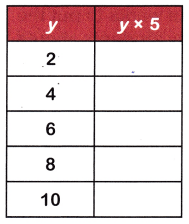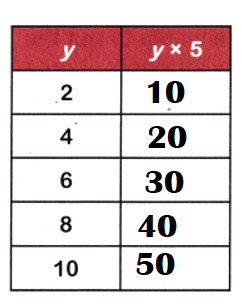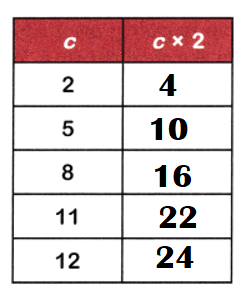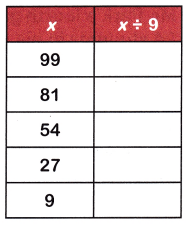Practice the questions of McGraw Hill Math Grade 4 Answer Key PDF Chapter 3 Test to secure good marks & knowledge in the exams.

Multiply.
Question 1.
10 × 4 = ____
Multiplying 10 by 4 we get the product 40.

Explanation:
Product of:
10 × 4 = 40.

Question 2.
9 × 5 = ___
Multiplying 9 by 5 we get the product 45.

Explanation:
Product of:
9 × 5 =45.

Question 3.
8 × 8 = ____
Multiplying 8 by 8 we get the product 64.

Explanation:
Product of:
8 × 8 = 64.

Question 4.
3 × 11 = ___
Multiplying 3 by 11 we get the product 33.

Explanation:
Product of:
3 × 11 = 33.

Question 5.
2 × 8 = ___
Multiplying 2 by 8 we get the product 16.

Explanation:
Product of:
2 × 8 = 16.

Question 6.
6 × 6 = ____
Multiplying 6 by 6 we get the product 36.

Explanation:
Product of:
6 × 6 = 36.

Question 7.
2 × 6 = ___
Multiplying 2 by 6 we get the product 12.

Explanation:
Product of:
2 × 6 = 12.

Question 8.
7 × 3 = ___
Multiplying 7 by 3 we get the product 21.

Explanation:
Product of:
7 × 3 = 21.

Question 9.
12 × 9 = ___
Multiplying 12 by 9 we get the product 108.

Explanation:
Product of:
12 × 9 = 108.

Divide.
Question 10.
48 ÷ 6 = ___
Dividing 48 by 6 we get quotient 8.

Explanation:
Quotient of:
48 ÷ 6 = 8.

Question 11.
81 ÷ 9 = __
Dividing 81 by 9 we get quotient 9.

Explanation:
Quotient of:
81 ÷ 9 = 9.

Question 12.
120 ÷ 10 = ___
Dividing 120 by 10 we get quotient 12.

Explanation:
Quotient of:
120 ÷ 10 = 12.

Question 13.
32 ÷ 8 = __
Dividing 32 by 8 we get quotient 4.

Explanation:
Quotient of:
32 ÷ 8 = 4.

Question 14.
9 ÷ 3 = ___
Dividing 9 by 3 we get quotient 3.

Explanation:
Quotient of:
9 ÷ 3 = 3.

Question 15.
24 ÷ 12 = ____
Dividing 24 by 12 we get quotient 2.

Explanation:
Quotient of:
24 ÷ 12 = 2.

Question 16.
63 ÷ 7 = ___
Dividing 63 by 7 we get quotient 9.

Explanation:
Quotient of:
63 ÷ 7 = 9.

Question 17.
28 ÷ 4 = ___
Dividing 28 by 4 we get quotient 7.

Explanation:
Quotient of:
28 ÷ 4 = 7.

Question 18.
25 ÷ 5 = ___
Dividing 25 by 5 we get quotient 5.

Explanation:
Quotient of:
25 ÷ 5 = 5.

Solve. Show the equation you used to find the answer.
Question 19.
Anna spent $40 on movie tickets for herself and her friends. She bought 8 tickets in all. How much does I ticket cost? Answer: Cost of each ticket =$5.

Explanation:
Cost of movie tickets Anna spent for herself and her friends = $40. Number of tickets she bought = 8. Cost of each ticket = Cost of movie tickets Anna spent for herself and her friends ÷ Number of tickets she bought =$40 ÷ 8
= \$5.

Question 20.
Mr. Park’s fourth grade class is going on a field trip. He wants to put the 24 students in his class into 6 equal groups. How many students should he put in each group?
Number of students he puts in each group = 4.

Explanation:
Number of students in his class = 24.
Number of groups = 6.
Number of students he puts in each group = Number of students in his class ÷ Number of groups
= 24 ÷ 6
= 4.

Question 21.
Jamie wants to count her button collection. She makes 7 rows with 5 buttons in each row. How many buttons does she have in all?
Number of buttons she have in all = 35.

Explanation:
Number of rows she makes = 7.
Number of buttons in each row = 5.
Number of buttons she have in all = Number of rows she makes × Number of buttons in each row
= 7 × 5
= 35.

Question 22.
Explain how knowing that 9 × 7 = 63 can help you solve 63 ÷ 7.
9 × 7 = 63.
=> 63 ÷ 7 = 9.

Explanation:
Product of:
9 × 7 = 63.
=> 63 ÷ 7 = 9.

Question 23.
Mitchell writes 4 × 5 to describe the position of the desks in the classroom. Catie writes 5 × 4. Who is correct? Explain.
Both are correct because rows × columns or columns × rows = same.

Explanation:
Product of:
4 × 5 = 20.
5 × 4 = 20.

Identify the property shown in each number sentence.
Question 24.
129 × 1 = 129
_________
129 × 1 = 129.
Multiplication property.

Explanation:
129 × 1 = 129.
=> Product of 129 × 1 = 129.

Question 25.
(8 + 4) × 5 = (8 × 5) + (4 × 5)
(8 + 4) × 5 = (8 × 5) + (4 × 5).
Distributive property strategy.

Explanation:
(8 + 4) × 5 = (8 × 5) + (4 × 5)
According to the distributive property, multiplying the sum of two or more addends by a number will give the same result as multiplying each addend individually by the number and then adding the products together.

Question 26.
(3 × 24) × 8 = 3 × (24 × 8)
(3 × 24) × 8 = 3 × (24 × 8)
Associative property of multiplication strategy.

Explanation:
(3 × 24) × 8 = 3 × (24 × 8)
This law simply states that with addition and multiplication of numbers, you can change the grouping of the numbers in the problem and it will not affect the answer.

Question 27.
8 × 50 = 50 × 8
8 × 50 = 50 × 8.
Commutative property of multiplication strategy.

Explanation:
8 × 50 = 50 × 8.
The commutative property is a math rule that says that the order in which we multiply numbers does not change the product.

Question 28.
102 × 25 = 25 × 102
102 × 25 = 25 × 102.
Commutative property of multiplication strategy.

Explanation:
102 × 25 = 25 × 102.
The commutative property is a math rule that says that the order in which we multiply numbers does not change the product.

Question 29.
15 × 9 = (10 × 9) + (5 × 9)
15 × 9 = (10 × 9) + (5 × 9)
Distributive property of multiplication.

Explanation:
15 × 9 = (10 × 9) + (5 × 9)
Because we are breaking apart the objects in each group. We need to add them back together to get the total product five times seven that’s five equal groups with seven objects in each group.

Use a multiplication property to solve.

Question 30.
(12 + 4) × 6 = ___
(12 + 4) × 6 = 96.

Explanation:
(12 + 4) × 6 = (12 × 6) + (4 × 6)
= 72 + 24
= 96.

Question 31.
404 × 1 = ___
404 × 1 = 404.

Explanation:
404 × 1 =1 × 404
= 404.

Use each rule to fill in the chart.
Question 32.Explanation:
y = 2
=> y × 5 = 2 × 5 = 10.
y = 4.
=> y × 5 = 4 × 5 = 20.
y = 6
=> y × 5 = 6 × 5 = 30.
y = 8
=> y × 5 = 8 × 5 = 40.
y = 10
=> y × 5 = 10 × 5 = 50.

Question 33.Explanation:
c = 2
=> c × 2 = 2 × 2 = 4.
c = 5
=> c × 2 = 5 × 2 = 10.
c = 8
=> c × 2 = 8 × 2 = 16.
c = 11
=> c × 2 = 11 × 2 = 22.
c = 12
=> c × 2 = 12 × 2 = 24.

Question 34.Explanation:
x = 99
=> x ÷ 9 = 99 ÷ 9 = 11.
x = 81
=> x ÷ 9 = 81 ÷ 9 = 9.
x = 54
=> x ÷ 9 = 54 ÷ 9 = 6.
x = 27
=> x ÷ 9 = 27 ÷ 9 = 3.
x = 9
=> x ÷ 9 = 9 ÷ 9 = 1.

Scroll to Top